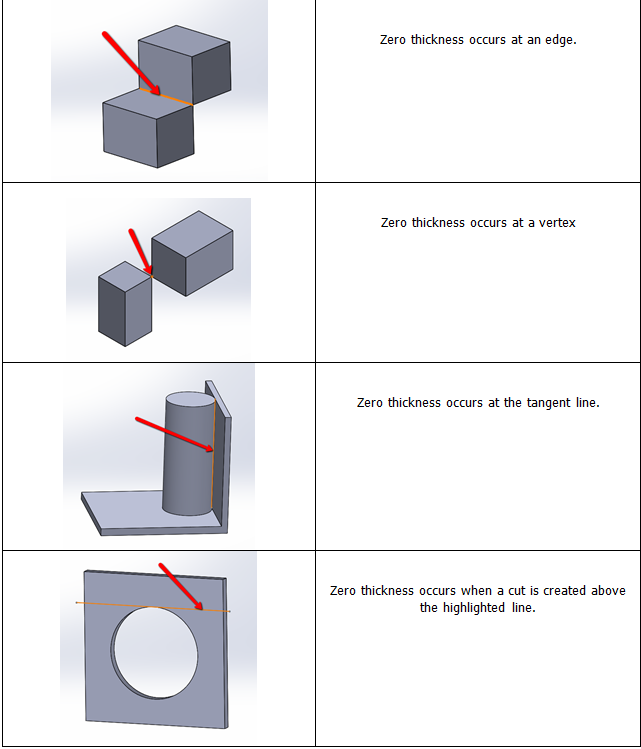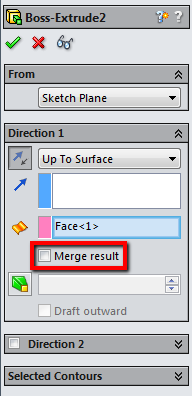# What is Zero Thickness Geometry?

While creating a model in SOLIDWORKS, some users run into zero thickness geometry errors, such as “Unable to create this feature because it would result in zero-thickness geometry” and “The model could not be properly sectioned by the section line. Please check that the section line cuts through the model”. This article describes the potential causes and solutions to zero thickness geometry error messages.

### Zero Thickness Geometry

Zero thickness geometry or non-manifold geometry occurs when edges or vertices of a solid model do not join with the adjacent geometry. SOLIDWORKS does not allow zero thickness geometry, due to mathematical problems and downstream errors in the model.

Examples of zero thickness geometry can be found below.One resolution is to add or remove enough solid material to the area of the zero thickness geometry to properly connect the edges and vertices.

Another solution is to disable the Merge result option in the Extrude PropertyManager.Understanding the cause of zero thickness geometry will lead to better designs by eliminating downstream errors in the model.